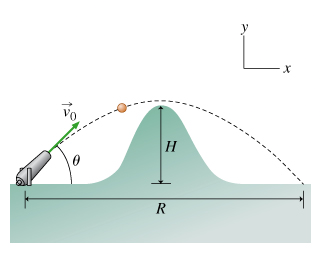# Problem:  A projectile is fired with speed v0 at an angle θ from the horizontal as shown in the figure below.Part A. Find the highest point in the trajectory, H. Express the highest point in terms of the magnitude of the acceleration due to gravity g, the initial velocity v0, and the angle θ.Part B. What is the range of the projectile, R? Express the range in terms of v0, θ, and g.

###### FREE Expert Solution

Kinematic equations:

Part A.

From the fourth kinematic equation:

87% (412 ratings)###### Problem Details

A projectile is fired with speed v0 at an angle θ from the horizontal as shown in the figure below.Part A. Find the highest point in the trajectory, H. Express the highest point in terms of the magnitude of the acceleration due to gravity g, the initial velocity v0, and the angle θ.

Part B. What is the range of the projectile, R? Express the range in terms of v0, θ, and g.